Young's Modulus Apparatus

Model: HO-ED-M-02

Holmarc's Young's modulus apparatus (Model No: HO-ED-M-02) is used to measure the Young's modulus of a bar. Young's modulus is a measure of stiffness of an elastic material and is a quantity used to characterize materials. It can vary considerably depending on the exact composition of the material. If the beam is loaded at its mid-point, the depression produced will not form an arc of a circle. This type of bending is called non-uniform bending. If the beam is loaded at both ends, the elevation produced will form an arc of a circle. This type of bending is called uniform bending. Two methods are used to measure Young's modulus of the bar in both uniform and non-uniform bending. They are Pin and Microscope method and Optic lever method.

In non uniform bending, the beam (meter scale) is supported symmetrically on two knife edges and loaded at its centre. The maximum depression is produced at its centre. Since the load is applied only at a point of the beam, this bending is not uniform through out the beam and the bending of the beam is called non-uniform bending.

Non uniform bendingIn uniform bending, the bar is placed symmetrically on two knife edges. Two weight hangers are suspended at equal distance from the knife edges. Weights are add one by one and corresponding readings are taken. From these readings, the mean elevation (e) of the midpoint of the bar for a given mass is determined.

Uniform bendingExperiment Examples

To find the Young's modulus of the material of a bar by uniform and non uniform bending using,

1. Pin and microscope method

1. Non uniform bending

Consider a bar of thickness d and breadth b is supported symmetrically between two knife edges at a distance l distance apart and loaded with a weight Mg at the center. The depression at the midpoint is given by,

Z = Mgl3 / 48 Y ( bd3 / 12 )

The Young’s Modulus of the material of the bar

Y = Mg (l3 / z) / 4bd3

For a constant mass M, the quantity l3/z is a constant from which Y can be calculated.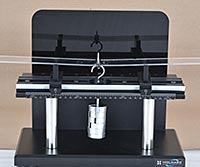2. Uniform bending

Consider a bar of thickness d and breadth b is supported symmetrically between two knife edges at a distance l distance apart and loaded with equal weights Mg at the ends at equal distance p from each knife edges. The elevation at the midpoint is given by,

Z = Mgpl2 / 8Y (bd3 / 12)

The Young’s Modulus of the material of the bar

Y = 3 Mgpl2 / 2bd3z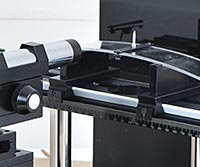2. Optic Lever Method

1. Non uniform bending

According to the theory of non uniform bending, for a bar of thickness d and breadth b ; supported by two knife edges l distance apart, the depression at the midpoint due to a load M is given by,

Z = Mgl3 / 48 Y (bd3 / 12)

The Young’s Modulus of the material of the bar

Y = Mg (l3 / z) / 4bd3

If the optical lever, scale and laser arrangement are used for measuring the depression, the angle of twist of optic lever

Θ = z / x

Where x is the perpendicular distance to the legs of the optical lever

If y to the shift on the scale arranged at a distance D from the laser of the optical lever then

Θ = y / 2D

Z = xy / 2D

Thus,

Y = Mg / 2bd3x (Dl3 / y)

For a mass M, the quantity Dl3/y is a constant.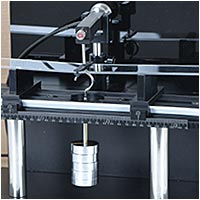2. Uniform bending

Consider a bar of thickness d and breadth b is supported symmetrically between two knife edges at a distance l distance apart and loaded with equal weights Mg at the ends at equal distance p from each knife edges. The elevation at the midpoint is given by,

Z = Mgpl2 / 8Y (bd3 / 12)

The Young’s Modulus of the material of the bar

Y = 3Mg pl2 / 2bd3z

If the optical lever, scale and laser arrangement are used for measuring the depression, the angle of twist of optic lever

Θ = z / x

Where x is the perpendicular distance to the legs of the optical lever

If y to the shift on the scale arranged at a distance D from the laser of the optical lever then

Θ = y / 2D

Z = xy / 2D

Thus,

Y = 3Mgpl2 / bd3xy)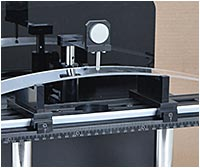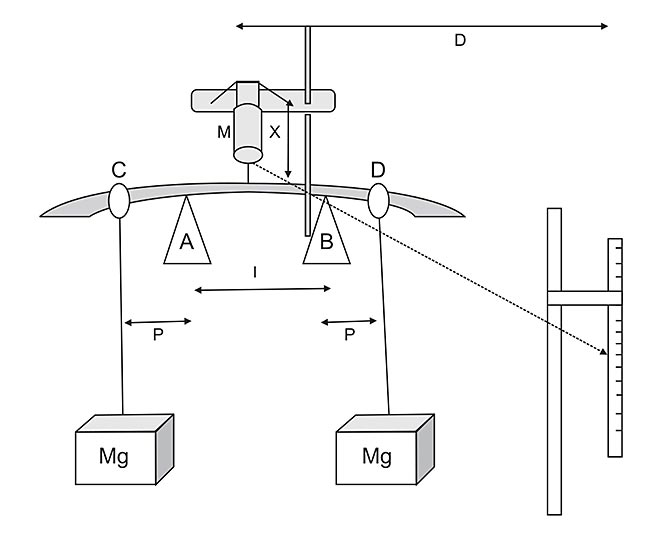Schematic Diagram

Related Topics

Young's modulus

Depression

Elevation

Bending - uniform and non uniform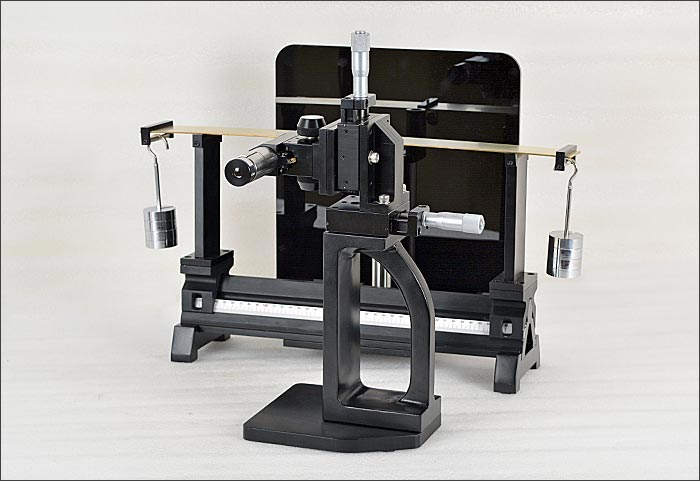Scope of Supply

Young's Modulus Test Bench

Model No: ED-M-02-YMTB
Material
:
Black Anodized Aluminum alloy
Quantity
:
1 no.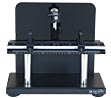Travelling Microscope

Model No: ED-M-02-TM
Magnification
:
10 X
Micrometer travel
:
25 mm
Least Count
:
0.01 mm
Quantity
:
1 no.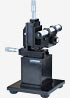Weight Hangers with Weights

Model No: ED-M-02-WHW
Material
:
Brass
Weights
:
100 gm & 50 gm
Quantity
:
2 Sets each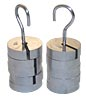Meter Scale with Rigid Base

Model No: ED-M-02-MSRB
Lengthl
:
1000 mm
Least Count
:
1 mm
Quantity
:
1 no.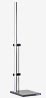Laser Line Generator with Power supply

Model No: ED-M-02-LLGP
Wave length
:
650 nm
Wave length
:
650 nm
Optical power
:
3 mW
Quantity
:
1 no.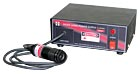Sample Test Plates

Model No: ED-M-02-STP
Material
:
Brass, Aluminium, Stainless steel, PMMA
Dimension
:
500 x 50 x 2 mm
Quantity
:
1no. each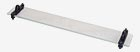Accessories

Allen Key
User Manual

|